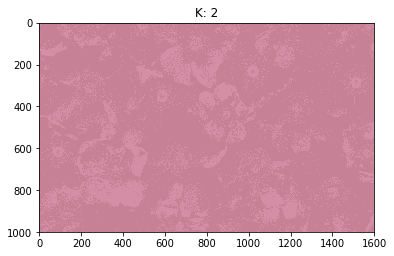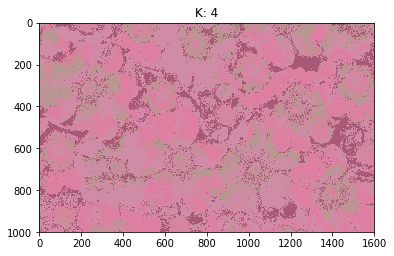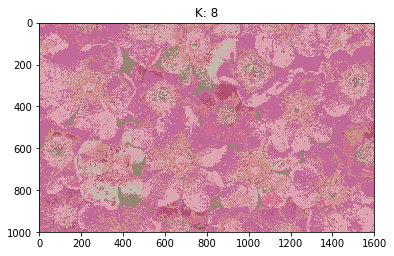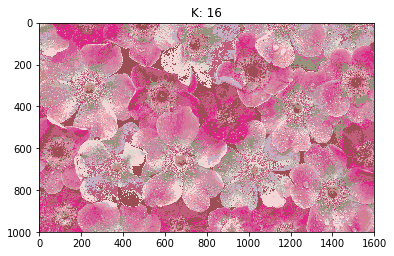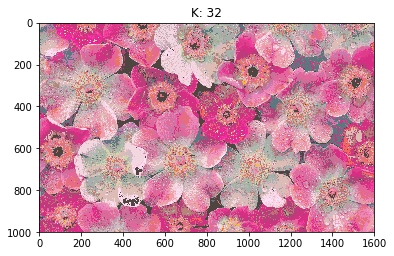# Clustering¶

Task: Given an image, cluster image pixels using partitional and hierarchical clustering algorithms.

In this project I have implemented k-means and agglomerative clustering algorithms. You will find brief explanations of my code throughout the implementation.

## K-means¶

K-means is basically an example of the Expectation-Maximization algorithm. Mainly, starting with randomly initialized cluster (mean) vectors $m_i$, we loop through:I used Mean Squared Error as my distance metric to evaluate pixel clusters in each iteration. Each cluster is represented with a mean vector, also called a centroid. Experimented with different k values and chose the optimal one using the elbow method.

### Code¶

Let's import the necessary libraries.

In :
import numpy as np
import scipy.io
import imageio
import pylab
import time
import matplotlib.pyplot as plt
from PIL import Image


I've created a class called Kmeans in order to have all the functionalities clean and neat. All the methods are explained as docstrings and comments in the code.

In :
class Kmeans:
def __init__(self, k):
self.K = k
self.num_of_K = len(k)
self.all_error = []

def init_rand_centroids(self, im, num_clusters):
""" Initialize centroids as random pixels in the image. Returns k-many random pixel RGB coordinates.

Steps in this method:

1. Create a 1-d numpy array that represents the indexes of each individual pixel in the input image
2. Shuffle the array
3. Use the shuffled array as a mask to obtain randomly selected centroids
4. Return the RGB values of the selected centroids
"""

initial_points = np.arange(im.shape)
np.random.shuffle(initial_points)
centroid_locs = im[initial_points[:num_clusters], :]
return centroid_locs

def update_centroids(self, mse, k, labels):
""" Returns new centroids using the current labeling of the pixels and the corresponding overall MSE.
"""
current_centroid = np.zeros((k, 3))
error = 0
for i in range(k):
indexes = np.where(labels == i)  # Indexes of pixel labels where they belong to ith cluster.
if len(indexes) != 0:
values = im2[indexes, :]  # Get the RGB values of the pixels using their indexes as mask.
current_centroid[i, :] = np.mean(values, axis=0)  # Compute the mean of the new centroid.
# Calculate MSE for the updated centroids.
error += np.sum((values[:, 0] - current_centroid[i, 0]).astype(np.float64) ** 2 +
(values[:, 1] - current_centroid[i, 1]).astype(np.float64) ** 2 +
(values[:, 2] - current_centroid[i, 2]).astype(np.float64) ** 2)
mse += error / len(labels)
return current_centroid, mse

def predict(self, k, new_im):
""" Return current labels of pixels, updated centroids, and corresponding MSE.
"""

# Select random centroids.
centroids = self.init_rand_centroids(new_im, k)

iteration = 0
mse = 0

# Compute distances of pixels to randomly selected centroids before the while loop as the first step.
dist = np.zeros((im2.shape, k))  # Distance matrix for each pixel.
for i in range(k):
dist[:, i] = np.linalg.norm(im2 - centroids[i], axis=1)  # This calculates the Euclidean distance.
labels = np.argmin(dist, axis=1)  # Assign each pixel to a cluster, which is the closest one.
centroids, mse = self.update_centroids(mse, k, labels)

# Repeat the above process until any pixels didn't change their labels.
while not (labels == np.argmin(dist, axis=1)).all():

if iteration != 0:
dist = np.zeros((im2.shape, k))
dist[:, i] = np.linalg.norm(im2 - centroids[i], axis=1)
labels = np.argmin(dist, axis=1)

centroids, mse = self.update_centroids(mse, k, labels)
iteration += 1

return labels, centroids, mse

def fit(self, im, im2):
""" Outputs the clustered image for each value of k.

im: Original image, of size (1000, 1600, 3) in this case.
im2: Reshaped image array from 3d to 2d. Thus in size (1600000, 3)
"""
clustered = np.zeros((self.num_of_K, im.shape, im.shape, 3), dtype=np.uint8)
err = []

# This loop is to run k-means algorithm for different given values of k.
# So it will run k-means algorithm as many as number of the k values provided (num_of_K).
for k in range(self.num_of_K):
print("Computing for K =", self.K[k])

labels, centroids, mse = self.predict(self.K[k], im2)
err.append(mse)  # Save mse values to plot them later.
labels = labels.reshape((im.shape, im.shape)).T  # Reshape label matrix back to original image dimensions.
clustered_im = np.zeros((im.shape, im.shape, 3), dtype=np.uint8)

# Re-construct the image with the final clustered pixels.
for i in range(im.shape):
for j in range(im.shape):
clustered_im[i, j, :] = centroids[labels[j, i], :].astype(np.uint8)

# Save clustered images for different k values to plot.
clustered[k, :, :, :] = clustered_im

print("Mean Squared Error = ", np.round(mse, decimals=2))
# Print centroids for k values smaller than 16 to prevent the longer output.
if self.K[k] <= 16:
print("Centroids =\n", np.around(centroids).astype(int))
print("------------------------")

# Plot the clustered images and the progressive MSE value.
for i in range(self.num_of_K):
plt.figure()
pylab.imshow(clustered[i, :, :, :])
pylab.title("K: {}".format(self.K[i]))

self.all_error.append(np.round(err[i], decimals=2))

plt.figure()
plt.plot(self.K, self.all_error)
plt.xlabel("K value")
plt.ylabel("Mean Squared Error")
plt.show()


Now we can read the image and run the algorithm with different k values. Centroid vectors, mean squared errors and the resulting images after clustering are reported in the output. As an example, I used k values (2, 4, 8, 16, 32, 64, 128).

In :
#%% Main

# Plot the original image.
plt.figure()
pylab.imshow(im)
pylab.title("Original Image")
plt.show()

# Convert to np array and reshape to work in 2d.
im = np.array(im)
im2 = im.reshape(-1, 3)
print('\nImage shape: {}.\n'.format(im.shape))

# Run k-means for the following k values.
K = (2, 4, 8, 16, 32, 64, 128)

start_time = time.time()

k_means = Kmeans(K)
k_means.fit(im, im2)
print("\nCluster vectors calculated for the K-values: ", K)
print("\nCorresponding MSE values: ", k_means.all_error)
print("\nTime passed: ", np.round((time.time() - start_time), decimals=2), "seconds.")
print("Process finished.")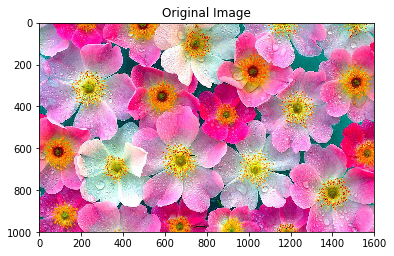Image shape: (1000, 1600, 3).

Computing for K = 2
Mean Squared Error =  10701.47
Centroids =
[[200 129 151]
[213 142 165]]
------------------------
Computing for K = 4
Mean Squared Error =  9797.66
Centroids =
[[209 141 167]
[181 153 142]
[169  90 119]
[223 128 161]]
------------------------
Computing for K = 8
Mean Squared Error =  9366.58
Centroids =
[[196 186 174]
[195 105 152]
[179  81 115]
[151 132 116]
[222 164 178]
[206 142 139]
[215 110 144]
[214 108 133]]
------------------------
Computing for K = 16
Mean Squared Error =  6753.66
Centroids =
[[196 178 200]
[222  39 136]
[189 143 162]
[237 169 180]
[196 179 177]
[206 143 164]
[157  79  85]
[193  94 124]
[150 147 126]
[215 112 154]
[245 213 214]
[230 143 169]
[223 216 209]
[202 137 137]
[220 117 160]
[210  85 148]]
------------------------
Computing for K = 32
Mean Squared Error =  5393.78
------------------------
Computing for K = 64
Mean Squared Error =  4662.58
------------------------
Computing for K = 128
Mean Squared Error =  2672.44
------------------------